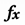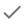You can use functions and formulas to automate calculations in Google Sheets.

Tip: If you’re already familiar with functions and formulas and just need to know which ones are available, go to Google Sheets function reference.

## Create a formula

2. Tap the cell where you want to add the formula.
• To pick from a list of functions, tap Function. Then choose a category and pick the function you want to use in your formula.
• To enter a function manually, enter `=` and the function you want to use.
3. A description of the function will show as you’re working on it to guide you through formula creation.
4. To use the content of other cells in your formula equation, type the names of the cells into the formula.
5. Choose from the symbols below the text box to add to the formula. For example, to subtract the contents of one cell from another, tap the - (minus) symbol.
6. To finish the formula, tap Done.

## Additional features for creating formulas

Nested functions

A function used in the same cell with another function is called a nested function. When functions are combined, Google Sheets will calculate the innermost function first. The nested function is contained in parentheses and is used as one of the components of the surrounding function.

For example, let's say you want to calculate the absolute value of a sum of several numbers in the cell range A1:A7. To calculate the sum of these numbers, you would enter '=SUM(A1:A7)' into a cell.

To calculate the absolute value of this sum, you need to nest the sum formula within absolute value formula. To calculate both formulas in a single cell, enter '=ABS(SUM(A1:A7))' into the cell. Note that the =SUM() function is performed first and is used as a component in the =ABS() function.

Formula highlighting

When you reference other cells in a formula, those cells will be highlighted in contrasting colors to help you more easily build a formula. When you click on a cell that contains a completed formula, you'll also see these cells highlighted.

## Functions that don't work

Some functions from other spreadsheet programs don't work in Sheets.
Type Description
CALL

Calls a dynamic link library or code resource. Since this resource might not be available on all devices, Sheets doesn’t use this function.

Tip: You can use macros or Apps Script instead.

CUBE functions (CUBEKPIMEMBER, CUBEMEMBER, CUBEMEMBERPROPERTY)

Lets you use Excel’s CUBE data model.

Tip: If you want to use similar CUBEs, you can use the Data Connectors feature.

INFO

Returns information about the Sheets document file, such as its filepath.

Note: Because Sheets emphasizes online collaboration, much of the information from this method may not be available or the most transparent to all users.

REGISTER.ID

Gets the registry ID from Windows.

Note: Since Sheets isn’t linked to any one operating system, this function isn’t supported.

RTD

Gets data from a Component Object Model (COM) Automation server.

Tip: Since everyone can’t get to the COM server, you can use macros or Apps Script.

WEBSERVICE

Relies on Windows to work fully.

Note: Since Sheets isn’t linked to any one operating system, this function isn’t supported.